# ML Aggarwal Solutions for Class 10 Maths Chapter 19 Trigonometric Tables

ML Aggarwal Solutions for Class 10 Maths Chapter 19 Trigonometric Tables, are provided here. Our solution module employs numerous shortcut tips and practical examples to explain all the exercise questions in a simple and easily understandable language. Our expert tutors at BYJU’S have prepared solutions with simpler steps which can be easily solved by students at ease. These solutions will help you in obtaining knowledge and strong command over the subject. Download pdf of ML Aggarwal Solutions for Class 10 Maths Chapter 19 in their respective links.

Chapter 19 – Trigonometric Tables. The approximate values correct up to four decimal places of sine, cosine and tangent of all angles lying between 0 degree and 90 degree can be found from the tables of natural sines, natural cosines and natural tangents given at the end of the book. These tables are called trigonometrical tables. In this ML Aggarwal Solutions for Class 10 Maths Chapter 19, contains the topics related to Trigonometric Tables and Use of Trigonometric Tables.

## Download the PDF of ML Aggarwal Solutions for Class 10 Maths Chapter 19 Trigonometric Tables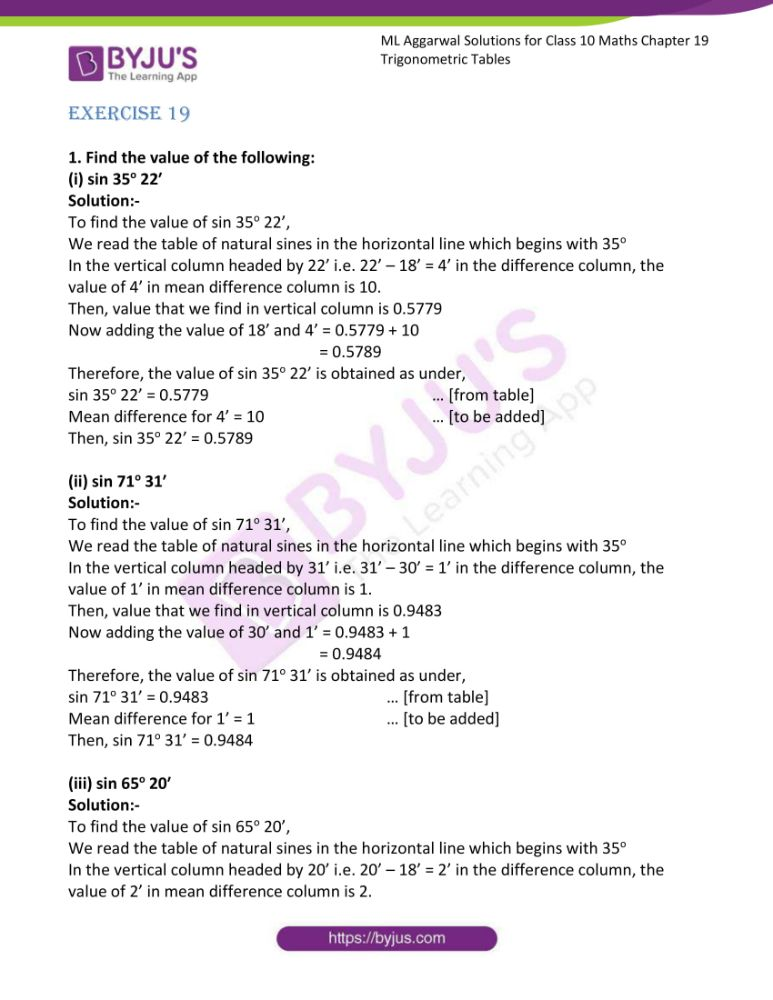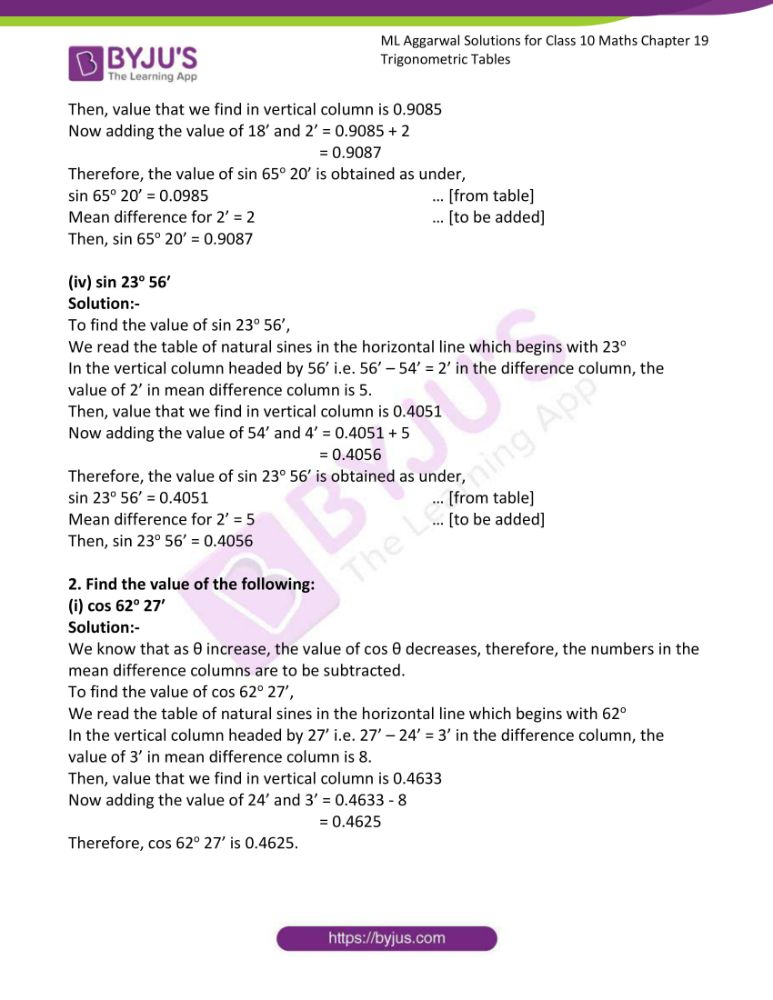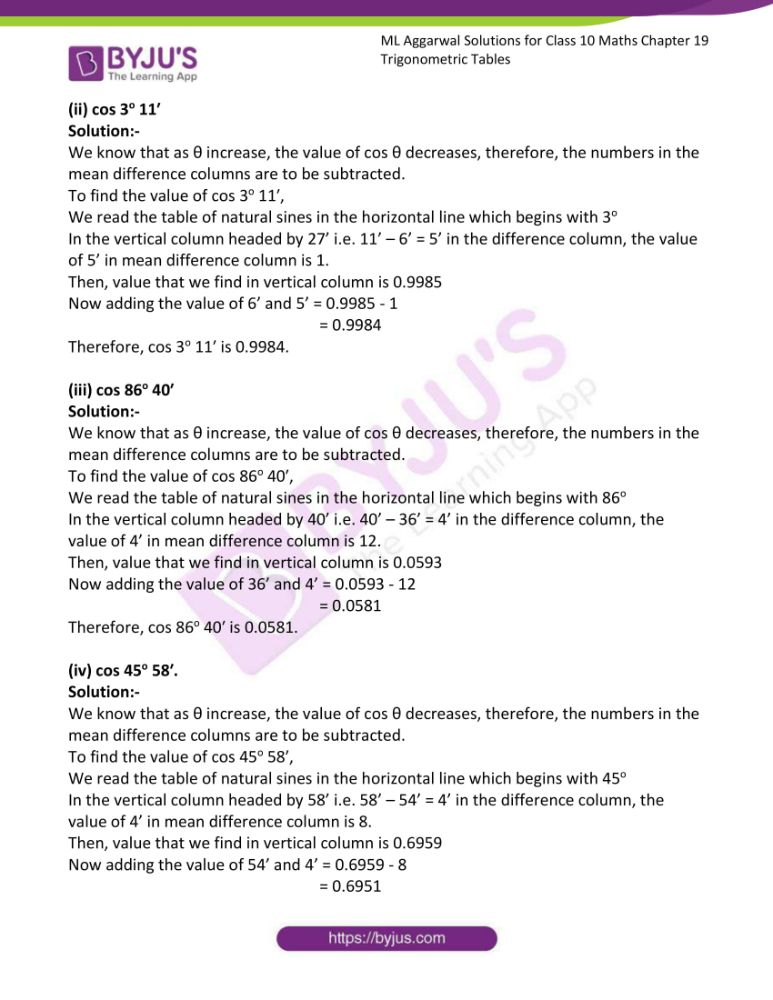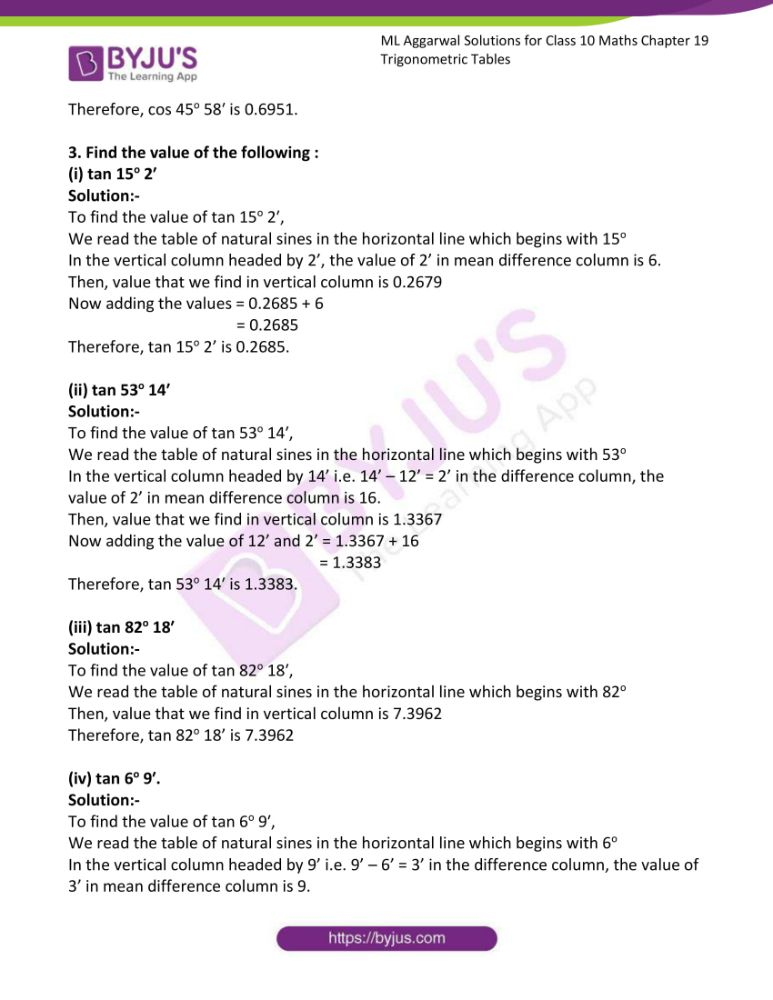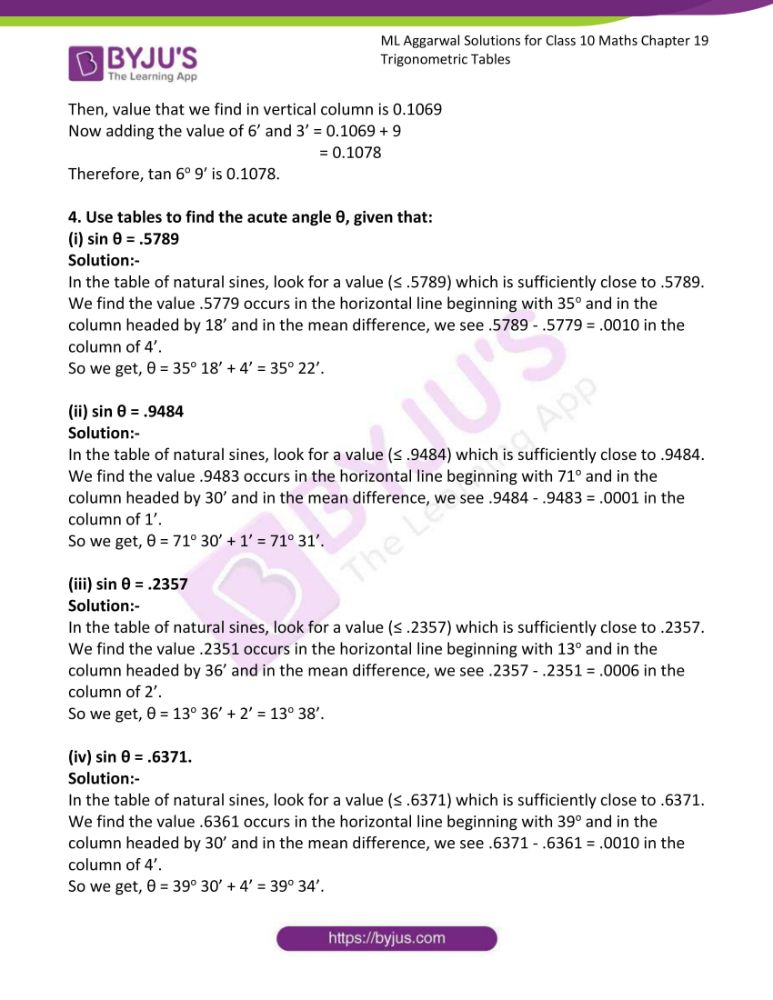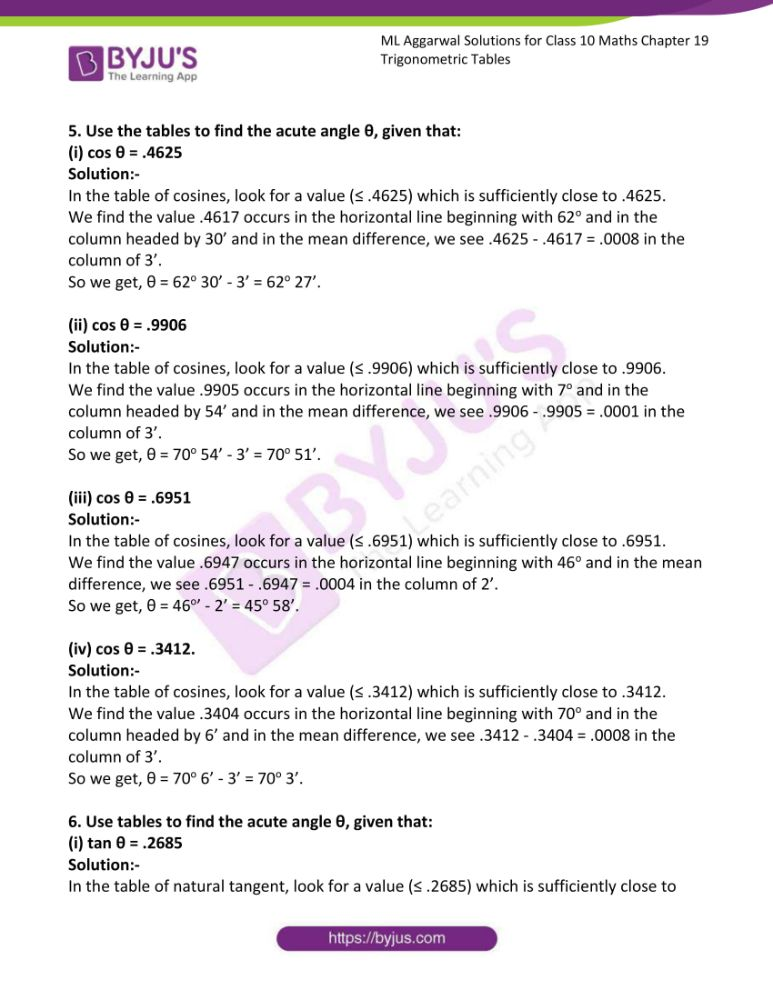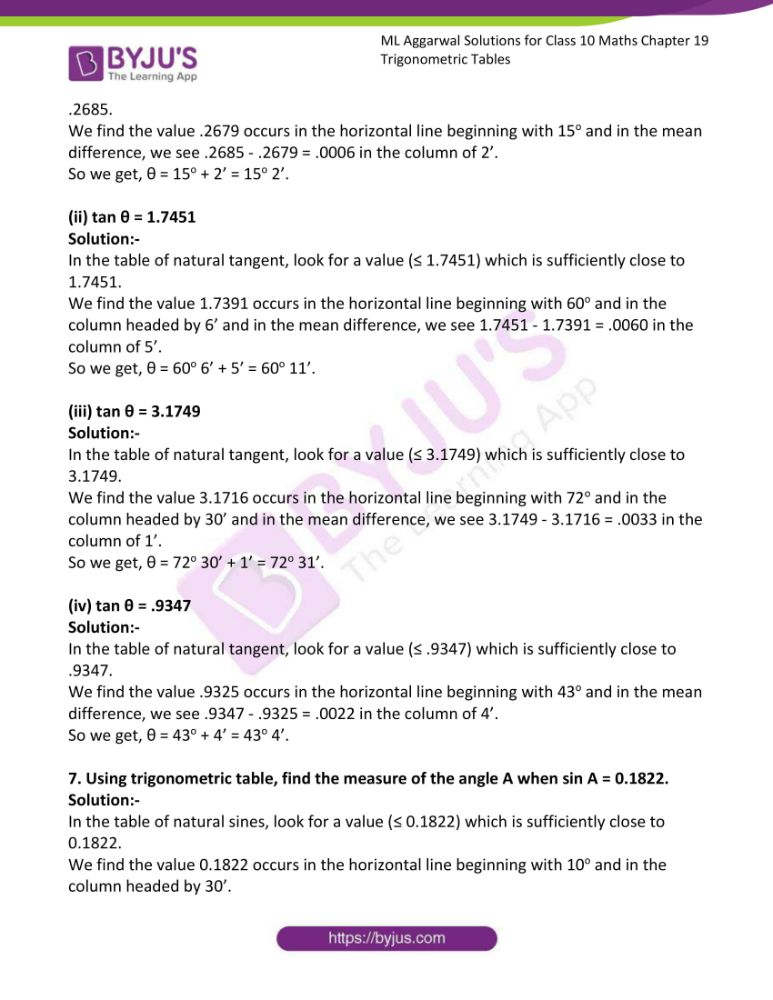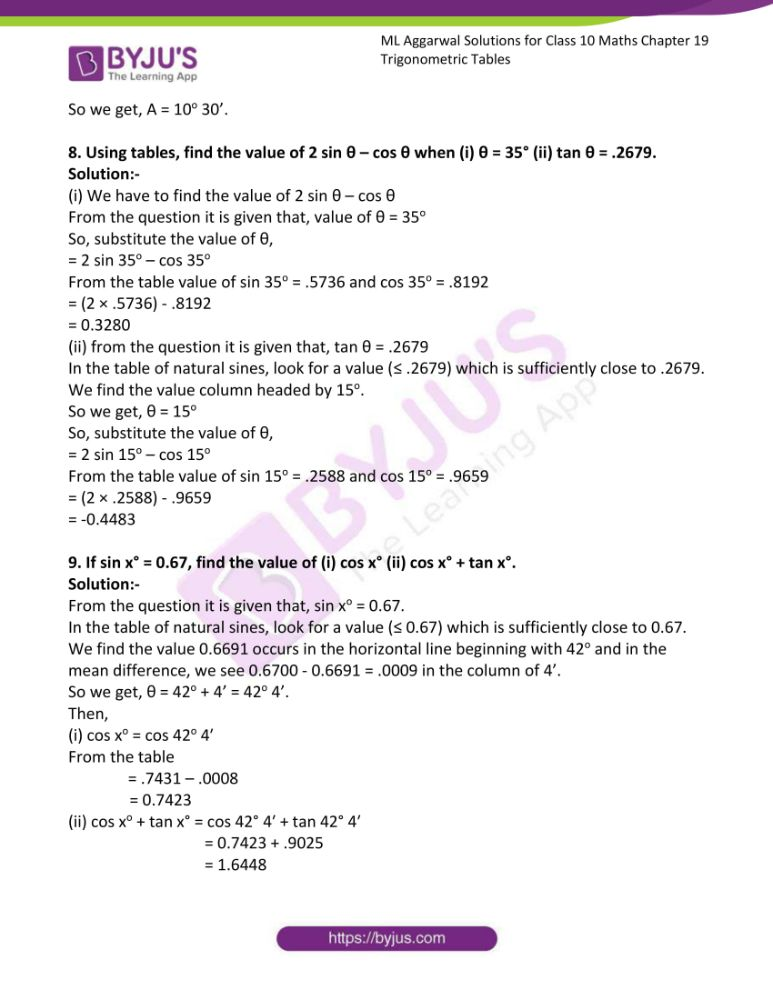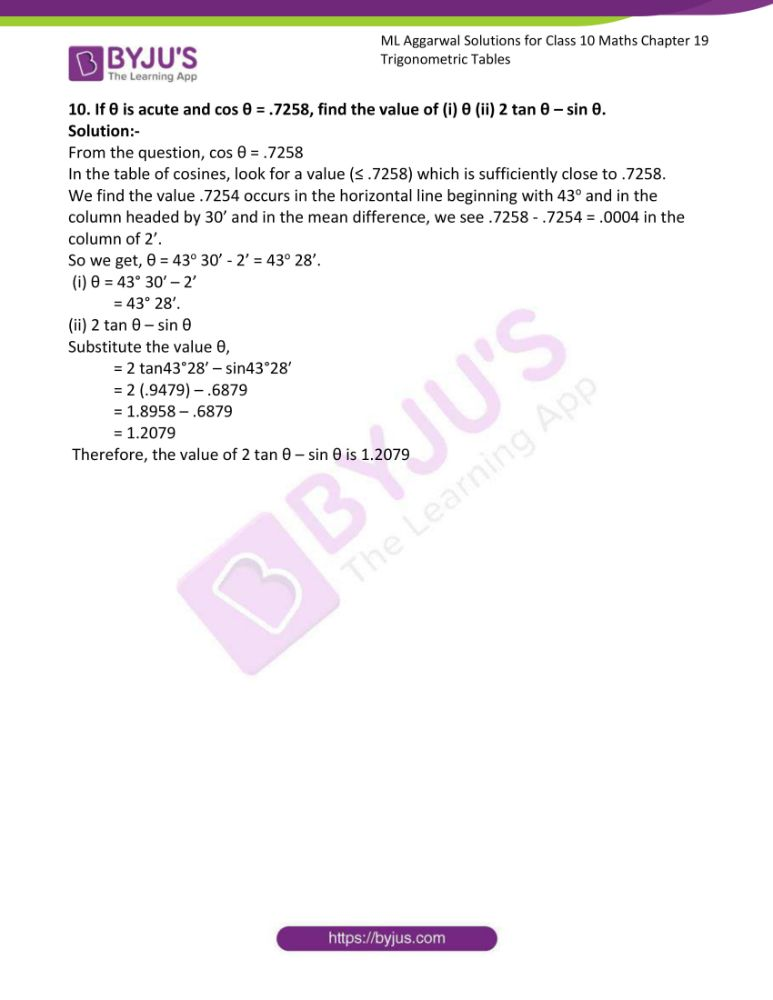### Access answers to ML Aggarwal Solutions for Class 10 Maths Chapter 19 Trigonometric Tables

Exercise 19

1. Find the value of the following:
(i) sin 35o 22′

Solution:-

To find the value of sin 35o 22’,

We read the table of natural sines in the horizontal line which begins with 35o

In the vertical column headed by 22’ i.e. 22’ – 18’ = 4’ in the difference column, the value of 4’ in mean difference column is 10.

Then, value that we find in vertical column is 0.5779

Now adding the value of 18’ and 4’ = 0.5779 + 10

= 0.5789

Therefore, the value of sin 35o 22’ is obtained as under,

sin 35o 22’ = 0.5779 … [from table]

Mean difference for 4’ = 10 … [to be added]

Then, sin 35o 22’ = 0.5789

(ii) sin 71o 31′

Solution:-

To find the value of sin 71o 31’,

We read the table of natural sines in the horizontal line which begins with 35o

In the vertical column headed by 31’ i.e. 31’ – 30’ = 1’ in the difference column, the value of 1’ in mean difference column is 1.

Then, value that we find in vertical column is 0.9483

Now adding the value of 30’ and 1’ = 0.9483 + 1

= 0.9484

Therefore, the value of sin 71o 31’ is obtained as under,

sin 71o 31’ = 0.9483 … [from table]

Mean difference for 1’ = 1 … [to be added]

Then, sin 71o 31’ = 0.9484

(iii) sin 65o 20′

Solution:-

To find the value of sin 65o 20’,

We read the table of natural sines in the horizontal line which begins with 35o

In the vertical column headed by 20’ i.e. 20’ – 18’ = 2’ in the difference column, the value of 2’ in mean difference column is 2.

Then, value that we find in vertical column is 0.9085

Now adding the value of 18’ and 2’ = 0.9085 + 2

= 0.9087

Therefore, the value of sin 65o 20’ is obtained as under,

sin 65o 20’ = 0.0985 … [from table]

Mean difference for 2’ = 2 … [to be added]

Then, sin 65o 20’ = 0.9087

(iv) sin 23o 56′

Solution:-

To find the value of sin 23o 56’,

We read the table of natural sines in the horizontal line which begins with 23o

In the vertical column headed by 56’ i.e. 56’ – 54’ = 2’ in the difference column, the value of 2’ in mean difference column is 5.

Then, value that we find in vertical column is 0.4051

Now adding the value of 54’ and 4’ = 0.4051 + 5

= 0.4056

Therefore, the value of sin 23o 56’ is obtained as under,

sin 23o 56’ = 0.4051 … [from table]

Mean difference for 2’ = 5 … [to be added]

Then, sin 23o 56’ = 0.4056

2. Find the value of the following:
(i) cos 62o 27′

Solution:-

We know that as θ increase, the value of cos θ decreases, therefore, the numbers in the mean difference columns are to be subtracted.

To find the value of cos 62o 27’,

We read the table of natural sines in the horizontal line which begins with 62o

In the vertical column headed by 27’ i.e. 27’ – 24’ = 3’ in the difference column, the value of 3’ in mean difference column is 8.

Then, value that we find in vertical column is 0.4633

Now adding the value of 24’ and 3’ = 0.4633 – 8

= 0.4625

Therefore, cos 62o 27’ is 0.4625.

(ii) cos 3o 11′

Solution:-

We know that as θ increase, the value of cos θ decreases, therefore, the numbers in the mean difference columns are to be subtracted.

To find the value of cos 3o 11′,

We read the table of natural sines in the horizontal line which begins with 3o

In the vertical column headed by 27’ i.e. 11’ – 6’ = 5’ in the difference column, the value of 5’ in mean difference column is 1.

Then, value that we find in vertical column is 0.9985

Now adding the value of 6’ and 5’ = 0.9985 – 1

= 0.9984

Therefore, cos 3o 11′ is 0.9984.

(iii) cos 86o 40′

Solution:-

We know that as θ increase, the value of cos θ decreases, therefore, the numbers in the mean difference columns are to be subtracted.

To find the value of cos 86o 40′,

We read the table of natural sines in the horizontal line which begins with 86o

In the vertical column headed by 40’ i.e. 40’ – 36’ = 4’ in the difference column, the value of 4’ in mean difference column is 12.

Then, value that we find in vertical column is 0.0593

Now adding the value of 36’ and 4’ = 0.0593 – 12

= 0.0581

Therefore, cos 86o 40′ is 0.0581.

(iv) cos 45o 58′.

Solution:-

We know that as θ increase, the value of cos θ decreases, therefore, the numbers in the mean difference columns are to be subtracted.

To find the value of cos 45o 58′,

We read the table of natural sines in the horizontal line which begins with 45o

In the vertical column headed by 58’ i.e. 58’ – 54’ = 4’ in the difference column, the value of 4’ in mean difference column is 8.

Then, value that we find in vertical column is 0.6959

Now adding the value of 54’ and 4’ = 0.6959 – 8

= 0.6951

Therefore, cos 45o 58′ is 0.6951.

3. Find the value of the following :
(i) tan 15o 2′

Solution:-

To find the value of tan 15o 2′,

We read the table of natural sines in the horizontal line which begins with 15o

In the vertical column headed by 2’, the value of 2’ in mean difference column is 6.

Then, value that we find in vertical column is 0.2679

Now adding the values = 0.2685 + 6

= 0.2685

Therefore, tan 15o 2’ is 0.2685.

(ii) tan 53o 14′

Solution:-

To find the value of tan 53o 14′,

We read the table of natural sines in the horizontal line which begins with 53o

In the vertical column headed by 14’ i.e. 14’ – 12’ = 2’ in the difference column, the value of 2’ in mean difference column is 16.

Then, value that we find in vertical column is 1.3367

Now adding the value of 12’ and 2’ = 1.3367 + 16

= 1.3383

Therefore, tan 53o 14′ is 1.3383.

(iii) tan 82o 18′

Solution:-

To find the value of tan 82o 18′,

We read the table of natural sines in the horizontal line which begins with 82o

Then, value that we find in vertical column is 7.3962

Therefore, tan 82o 18’ is 7.3962

(iv) tan 6o 9′.

Solution:-

To find the value of tan 6o 9′,

We read the table of natural sines in the horizontal line which begins with 6o

In the vertical column headed by 9’ i.e. 9’ – 6’ = 3’ in the difference column, the value of 3’ in mean difference column is 9.

Then, value that we find in vertical column is 0.1069

Now adding the value of 6’ and 3’ = 0.1069 + 9

= 0.1078

Therefore, tan 6o 9′ is 0.1078.

4. Use tables to find the acute angle θ, given that:
(i) sin θ = .5789

Solution:-

In the table of natural sines, look for a value (≤ .5789) which is sufficiently close to .5789.

We find the value .5779 occurs in the horizontal line beginning with 35o and in the column headed by 18’ and in the mean difference, we see .5789 – .5779 = .0010 in the column of 4’.

So we get, θ = 35o 18’ + 4’ = 35o 22’.

(ii) sin θ = .9484

Solution:-

In the table of natural sines, look for a value (≤ .9484) which is sufficiently close to .9484.

We find the value .9483 occurs in the horizontal line beginning with 71o and in the column headed by 30’ and in the mean difference, we see .9484 – .9483 = .0001 in the column of 1’.

So we get, θ = 71o 30’ + 1’ = 71o 31’.

(iii) sin θ = .2357

Solution:-

In the table of natural sines, look for a value (≤ .2357) which is sufficiently close to .2357.

We find the value .2351 occurs in the horizontal line beginning with 13o and in the column headed by 36’ and in the mean difference, we see .2357 – .2351 = .0006 in the column of 2’.

So we get, θ = 13o 36’ + 2’ = 13o 38’.

(iv) sin θ = .6371.

Solution:-

In the table of natural sines, look for a value (≤ .6371) which is sufficiently close to .6371.

We find the value .6361 occurs in the horizontal line beginning with 39o and in the column headed by 30’ and in the mean difference, we see .6371 – .6361 = .0010 in the column of 4’.

So we get, θ = 39o 30’ + 4’ = 39o 34’.

5. Use the tables to find the acute angle θ, given that:

(i) cos θ = .4625

Solution:-

In the table of cosines, look for a value (≤ .4625) which is sufficiently close to .4625.

We find the value .4617 occurs in the horizontal line beginning with 62o and in the column headed by 30’ and in the mean difference, we see .4625 – .4617 = .0008 in the column of 3’.

So we get, θ = 62o 30’ – 3’ = 62o 27’.

(ii) cos θ = .9906

Solution:-

In the table of cosines, look for a value (≤ .9906) which is sufficiently close to .9906.

We find the value .9905 occurs in the horizontal line beginning with 7o and in the column headed by 54’ and in the mean difference, we see .9906 – .9905 = .0001 in the column of 3’.

So we get, θ = 70o 54’ – 3’ = 70o 51’.

(iii) cos θ = .6951

Solution:-

In the table of cosines, look for a value (≤ .6951) which is sufficiently close to .6951.

We find the value .6947 occurs in the horizontal line beginning with 46o and in the mean difference, we see .6951 – .6947 = .0004 in the column of 2’.

So we get, θ = 46o’ – 2’ = 45o 58’.

(iv) cos θ = .3412.

Solution:-

In the table of cosines, look for a value (≤ .3412) which is sufficiently close to .3412.

We find the value .3404 occurs in the horizontal line beginning with 70o and in the column headed by 6’ and in the mean difference, we see .3412 – .3404 = .0008 in the column of 3’.

So we get, θ = 70o 6’ – 3’ = 70o 3’.

6. Use tables to find the acute angle θ, given that:

(i) tan θ = .2685

Solution:-

In the table of natural tangent, look for a value (≤ .2685) which is sufficiently close to .2685.

We find the value .2679 occurs in the horizontal line beginning with 15o and in the mean difference, we see .2685 – .2679 = .0006 in the column of 2’.

So we get, θ = 15o + 2’ = 15o 2’.

(ii) tan θ = 1.7451

Solution:-

In the table of natural tangent, look for a value (≤ 1.7451) which is sufficiently close to 1.7451.

We find the value 1.7391 occurs in the horizontal line beginning with 60o and in the column headed by 6’ and in the mean difference, we see 1.7451 – 1.7391 = .0060 in the column of 5’.

So we get, θ = 60o 6’ + 5’ = 60o 11’.

(iii) tan θ = 3.1749

Solution:-

In the table of natural tangent, look for a value (≤ 3.1749) which is sufficiently close to 3.1749.

We find the value 3.1716 occurs in the horizontal line beginning with 72o and in the column headed by 30’ and in the mean difference, we see 3.1749 – 3.1716 = .0033 in the column of 1’.

So we get, θ = 72o 30’ + 1’ = 72o 31’.

(iv) tan θ = .9347

Solution:-

In the table of natural tangent, look for a value (≤ .9347) which is sufficiently close to .9347.

We find the value .9325 occurs in the horizontal line beginning with 43o and in the mean difference, we see .9347 – .9325 = .0022 in the column of 4’.

So we get, θ = 43o + 4’ = 43o 4’.

7. Using trigonometric table, find the measure of the angle A when sin A = 0.1822.

Solution:-

In the table of natural sines, look for a value (≤ 0.1822) which is sufficiently close to 0.1822.

We find the value 0.1822 occurs in the horizontal line beginning with 10o and in the column headed by 30’.

So we get, A = 10o 30’.

8. Using tables, find the value of 2 sin θ – cos θ when (i) θ = 35° (ii) tan θ = .2679.

Solution:-

(i) We have to find the value of 2 sin θ – cos θ

From the question it is given that, value of θ = 35o

So, substitute the value of θ,

= 2 sin 35o – cos 35o

From the table value of sin 35o = .5736 and cos 35o = .8192

= (2 × .5736) – .8192

= 0.3280

(ii) from the question it is given that, tan θ = .2679

In the table of natural sines, look for a value (≤ .2679) which is sufficiently close to .2679.

We find the value column headed by 15o.

So we get, θ = 15o

So, substitute the value of θ,

= 2 sin 15o – cos 15o

From the table value of sin 15o = .2588 and cos 15o = .9659

= (2 × .2588) – .9659

= -0.4483

9. If sin x° = 0.67, find the value of (i) cos x° (ii) cos x° + tan x°.

Solution:-

From the question it is given that, sin xo = 0.67.

In the table of natural sines, look for a value (≤ 0.67) which is sufficiently close to 0.67.

We find the value 0.6691 occurs in the horizontal line beginning with 42o and in the mean difference, we see 0.6700 – 0.6691 = .0009 in the column of 4’.

So we get, θ = 42o + 4’ = 42o 4’.

Then,

(i) cos xo = cos 42o 4′

From the table

= .7431 – .0008

= 0.7423

(ii) cos xo + tan x° = cos 42° 4′ + tan 42° 4′

= 0.7423 + .9025

= 1.6448

10. If θ is acute and cos θ = .7258, find the value of (i) θ (ii) 2 tan θ – sin θ.

Solution:-

From the question, cos θ = .7258

In the table of cosines, look for a value (≤ .7258) which is sufficiently close to .7258.

We find the value .7254 occurs in the horizontal line beginning with 43o and in the column headed by 30’ and in the mean difference, we see .7258 – .7254 = .0004 in the column of 2’.

So we get, θ = 43o 30’ – 2’ = 43o 28’.

(i) θ = 43° 30′ – 2’

= 43° 28′.

(ii) 2 tan θ – sin θ

Substitute the value θ,

= 2 tan43°28′ – sin43°28′

= 2 (.9479) – .6879

= 1.8958 – .6879

= 1.2079

Therefore, the value of 2 tan θ – sin θ is 1.2079